edraw-max-horizontal
Product Tour
Templates
Guide
What's New
Start Now

# Examples for Algorithm Flowcharts

> Examples for Algorithm Flowcharts
Posted by Allison Lynch | 07/21/2021

#### Part 1: Algorithm and flowchart

Algorithms and flowcharts are two different ways of presenting the process of solving a problem. Algorithms consist of steps for solving a particular problem, while in flowcharts, those steps are usually displayed in shapes and process boxes with arrows. So flowcharts can be used for presenting algorithms. This page will introduce some examples of algorithm flowcharts.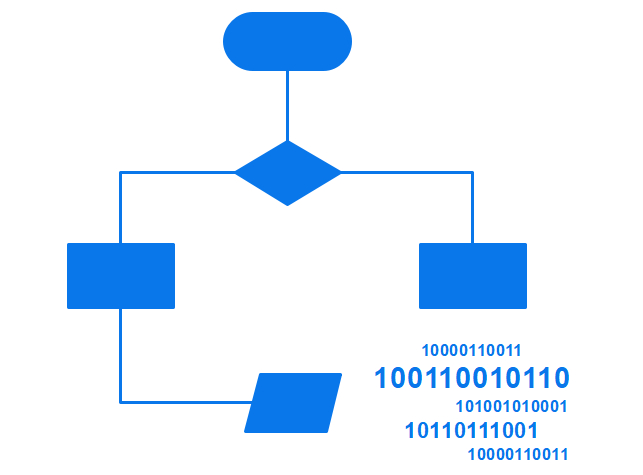#### Part 2: Rules of Drawing Flowcharts for Algorithms

Flowcharts usually consist of basic symbols and shapes that have a specific representation. The symbols make the chart more meaningful and easy to understand.

Some of the most standard symbols include shapes like a rectangle representing an operation, a parallelogram showing input and output of data, a cylinder indicating the start/end, while a diamond represents a decision. Read more about flowchart symbols.

However, knowing these symbols well and using them in a flowchart involves critical thinking. Here are some basic rules that need to be considered while drawing a flowchart.

1. All the boxes and shapes in a flowchart should be connected with arrows to indicate a logical order and make it easy to follow.
2. Make sure the flow direction is consistent and not ambiguous. The flow charts always flow from either left to right or top to bottom.
3. Every chart will begin with a Start box and end with a Terminal box, with the latter only having one flow line associated with it.
4. All the shapes, symbols, and lines should be consistent. You can also add a symbol key to make it easier to follow the chart.

## EdrawMax

##### All-in-One Diagram Software
Create more than 280 types of diagrams effortlessly
Start diagramming with various templates and symbols easily
• Superior file compatibility: Import and export drawings to various file formats, such as Visio
• Cross-platform supported (Windows, Mac, Linux, Web)Security Verified | Switch to Mac >>

#### Part 3: Algorithm and Flowchart Examples

##### Example 1: Determine and Output Whether Number N is Even or Odd
• Step 1: Read number N.
• Step 2: Set remainder as N modulo 2.
• Step 3: If the remainder is equal to 0 then number N is even, else number N is odd.
• Step 4: Print output.##### Example 2: Calculate the Interest of a Bank Deposit
• Step 4: Calculate the interest with the formula "Interest=Amount*Years*Rate/100.
• Step 5: Print interest.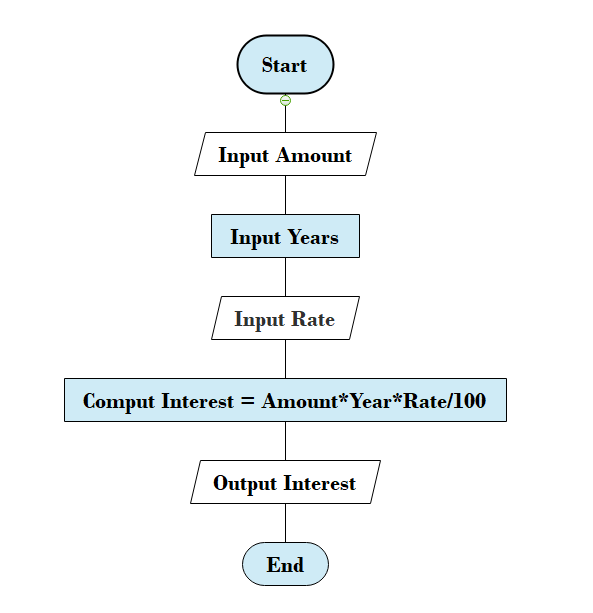##### Example 3: Determine Whether a Temperature is Below or Above the Freezing Point
• Step 1: Input temperature.
• Step 2: If it is less than 32, then print "below freezing point", otherwise print "above freezing point".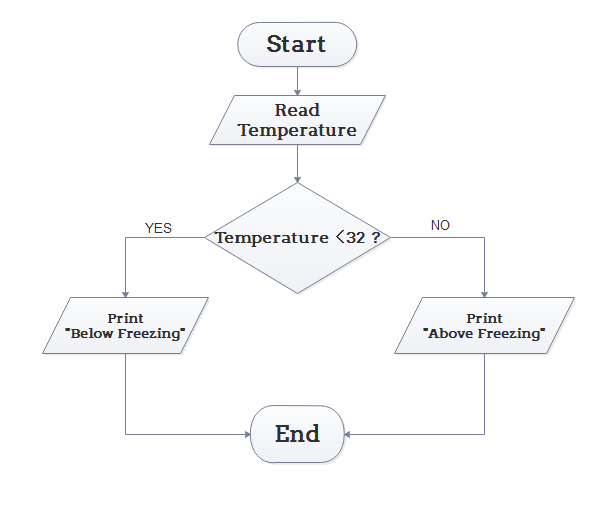##### Example 4: Determine Whether A Student Passed the Exam or Not
• Step 1: Input grades of 4 courses M1, M2, M3 and M4.
• Step 3: If the average grade is less than 60, print "FAIL", else print "PASS".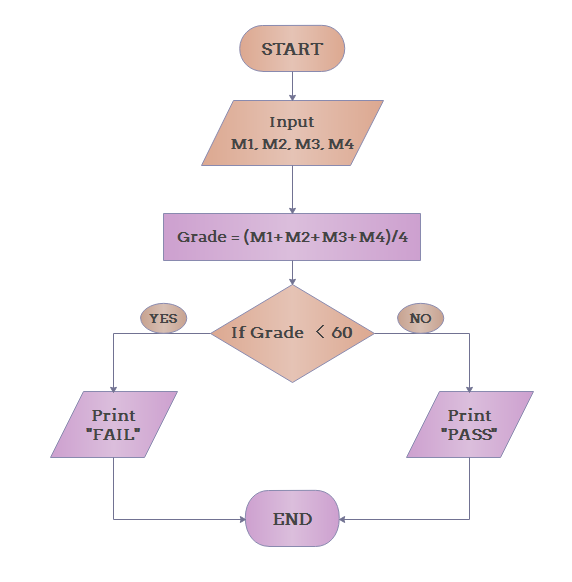##### Example 5: Find the Sum of Two Numbers Entered
• Step 1: Read the Integer A.
• Step 2: Read Integer B.
• Step 3: Perform the addition by using the formula: C= A + B.
• Step 4: Print the Integer C.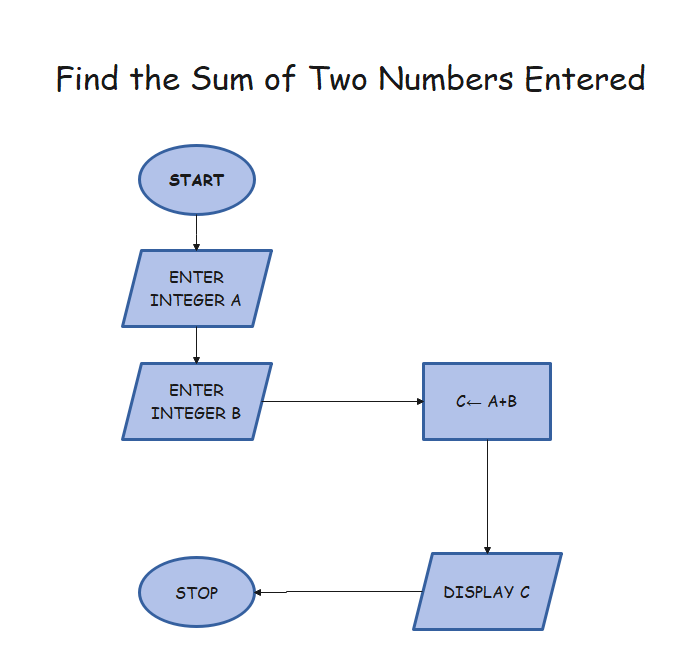##### Example 6: Determining the Largest Number Among All the Entered Integers
• Step 1: Read the Integer A.
• Step 2: Read Integer B.
• Step 3: If B is greater than A, then print B, else A.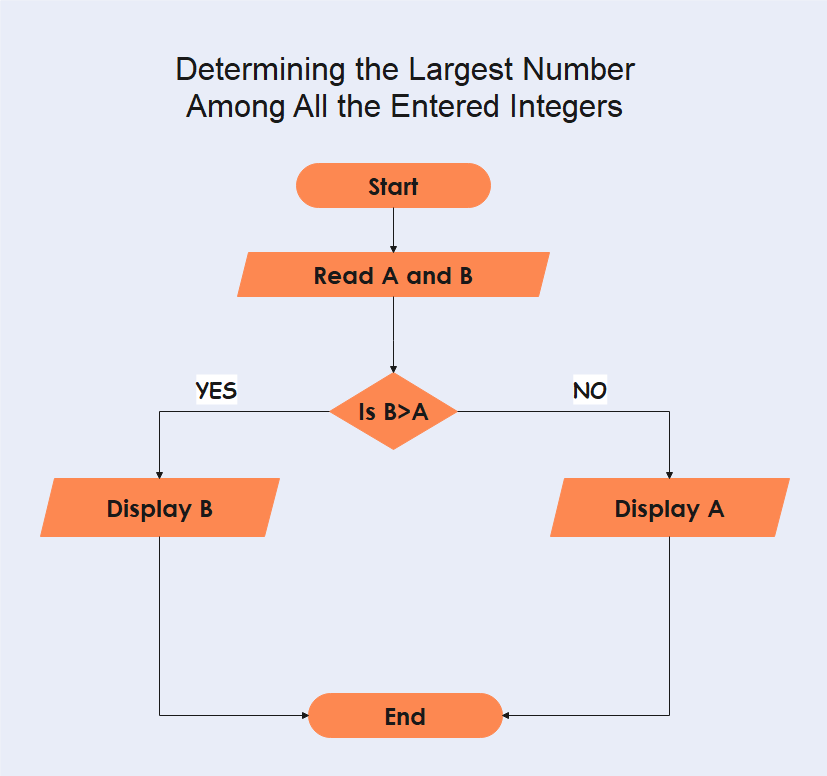##### Example 7: Work Out All the Roots of a Quadratic Equation ax² +bx+c=0
• Step 1: Enter the variables a, b, c, D, x1, x2, rp, and ip.
• Step 2: Evaluate the discriminant by using the formula: D= b²- 4ac
• Step 3: Print rp and ip.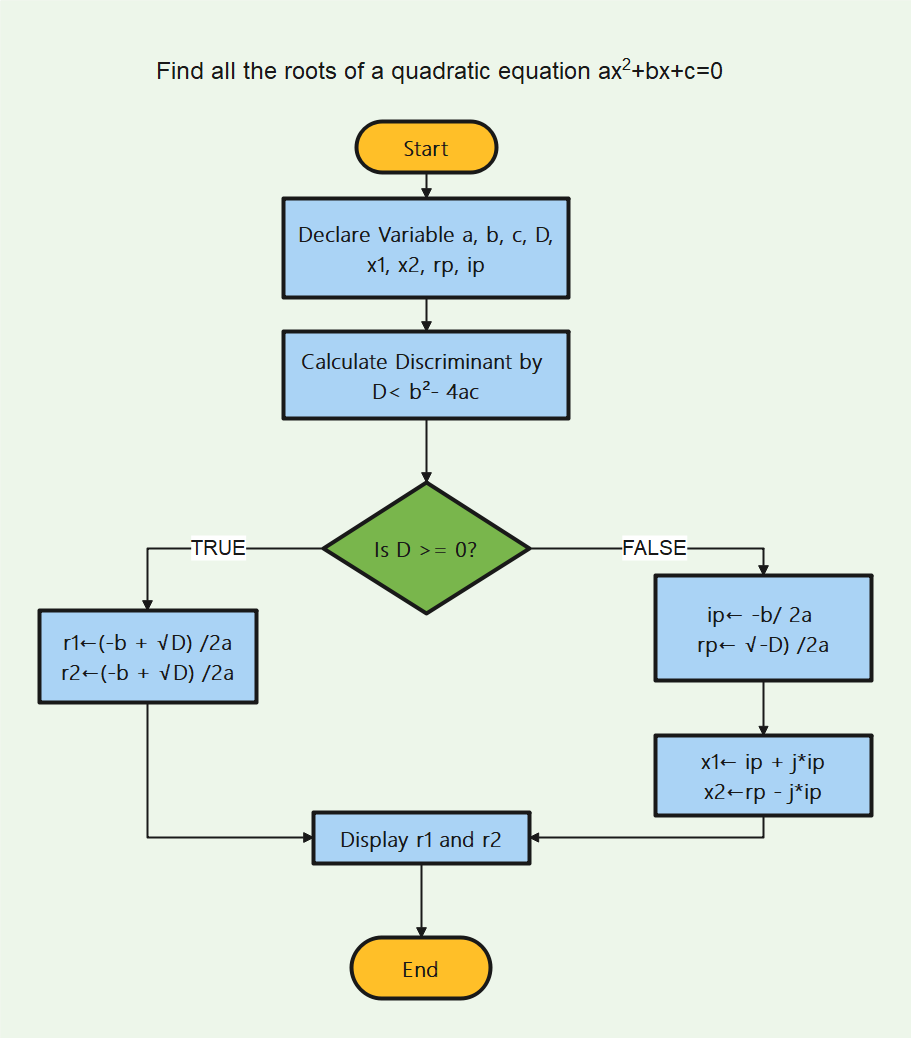##### Example 8: Find the Fibonacci series till term ≤1000
• Step 1: Declare the variables i, a, b, show.
• Step 2: Enter the values for the variables, a=0, b=1, show=0
• Step 3: Enter the terms of the Fibonacci series to be printed, i.e=, 1000.
• Step 4: Print the first two terms of the series.
• Step 5: Loop the following steps:
• Show = a + b
• a= b
• b = show
• Add 1 to the value of i each time.
• Print Show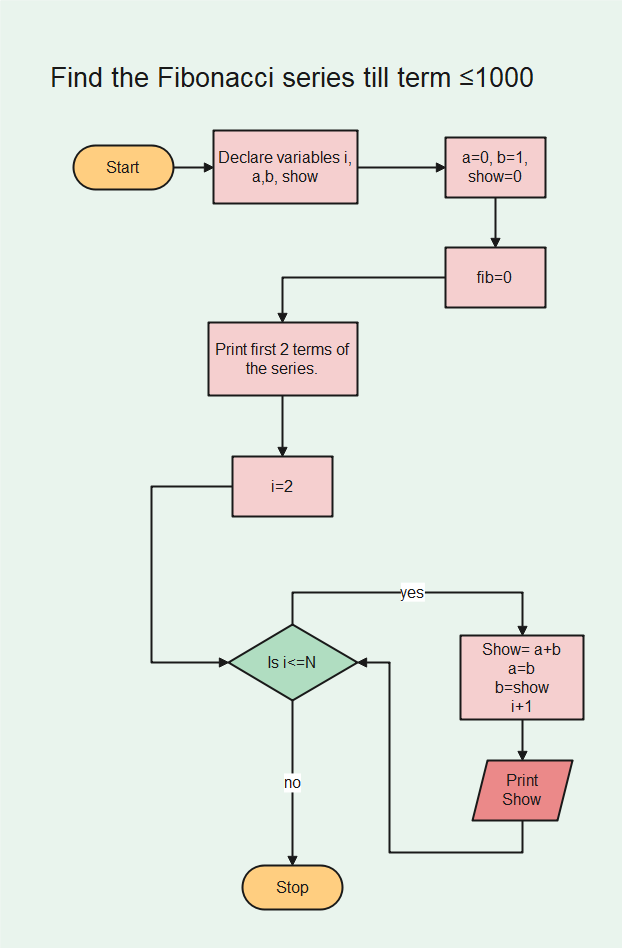##### Example 9: Calculate the Sum of The First 50 Numbers
• Step 1: Declare number N= 0 and sum= 0
• Step 2: Determine N by N= N+1
• Step 3: Calculate the sum by the formula: Sum= N + Sum.
• Step 4: Add a loop between steps 2 and 3 until N= 50.
• Step 5: Print Sum.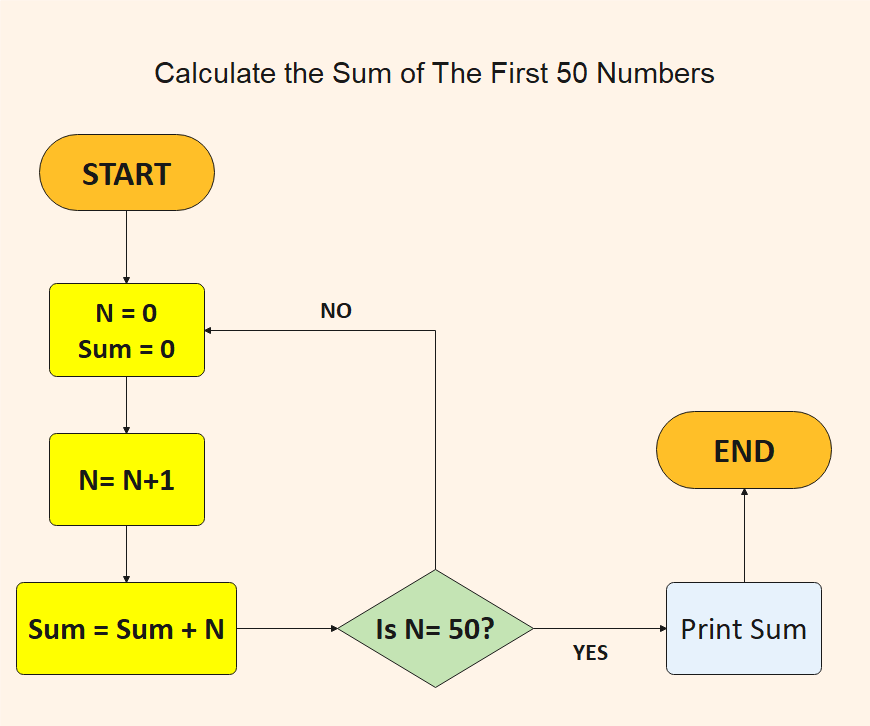##### Example 10: Find the largest price among 100 given values and reduce it by 10%
• Step 1: Read the 100 prices.
• Step 2: Compare the first price with the next and let the greater of the two be ‘max’ in the ‘max index.’
• Step 3: Loop it until the largest price has been found.
• Step 4: Reduce the ‘max’ value by 10% using the formula: prices [max index] = prices [max index] x 0.9.
• Step 5: Print.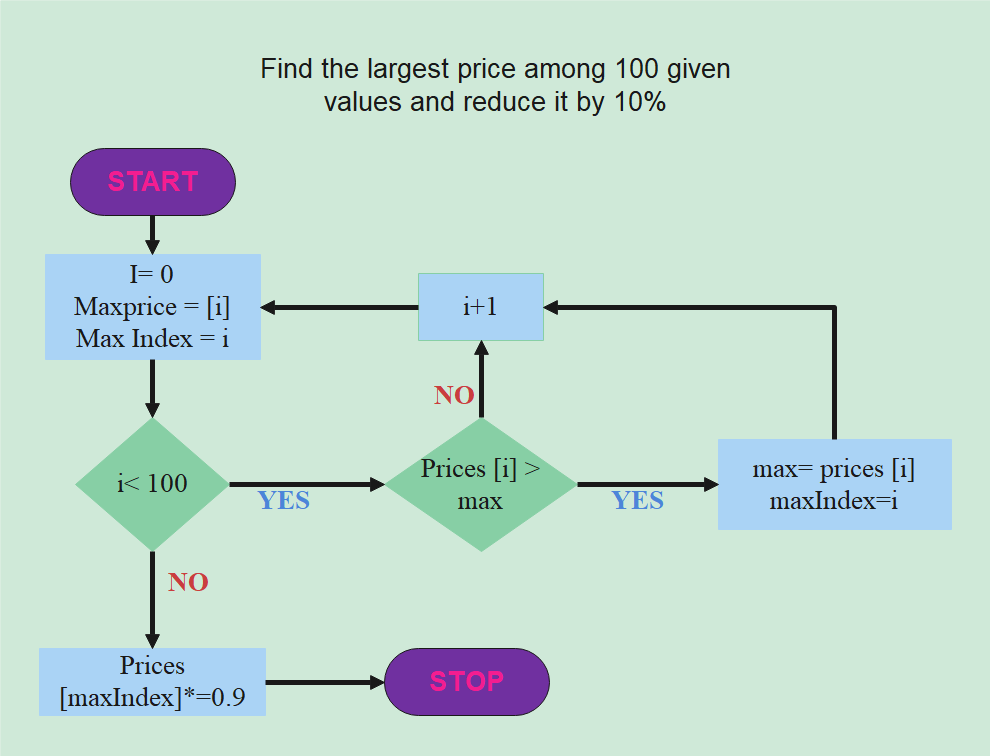##### Example 11: Arrange the numbers X, Y, Z in descending order
• Step 1: Input the value for variables X, Y, Z.
• Step 2: Read the integers X, Y, Z.
• Step 3: Check if x>y, x>z, y>z
• Step 4: Print the possible order.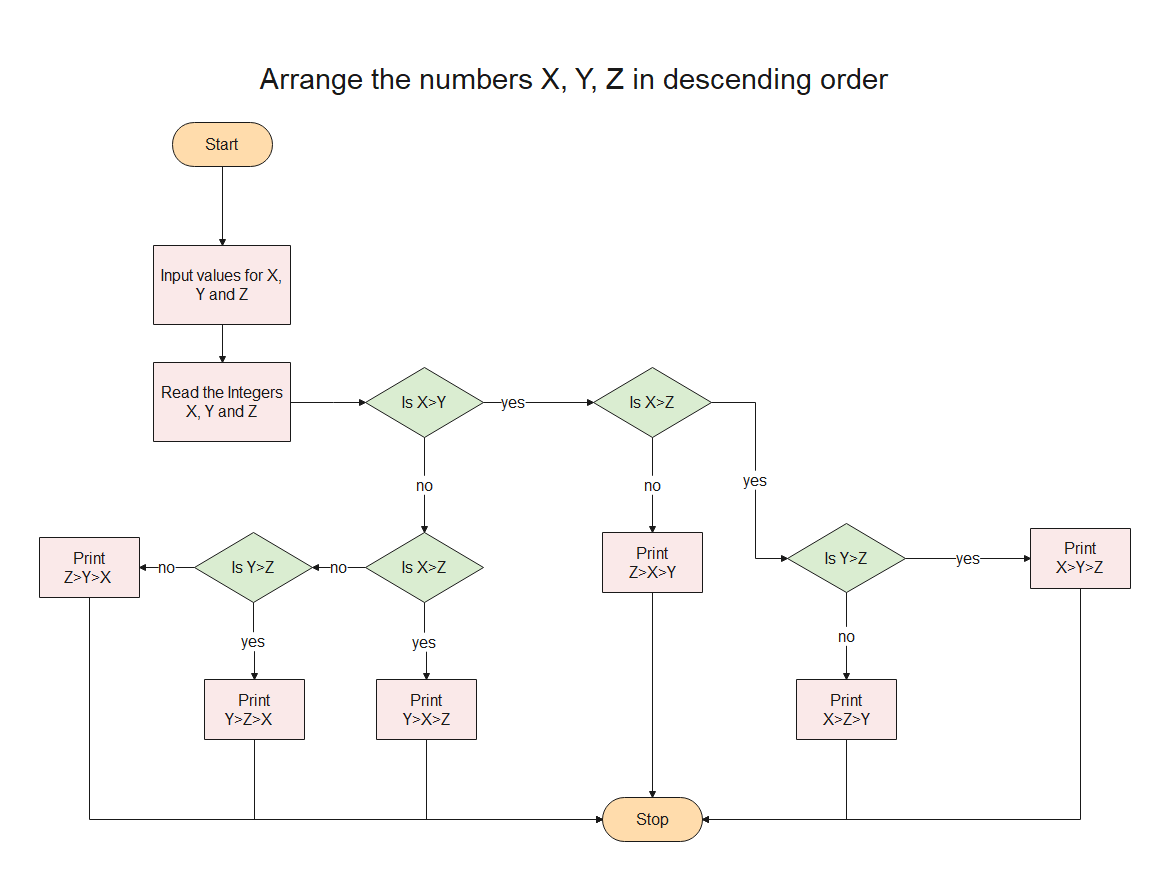##### Example 12: Determine the Highest Common Factor (HCF) of two integers
• Step 1: Read the variables a and b.
• Step 2: If a = b, go to step 4.
• Step 3: If a > b, then: a = a - b. Return to step 2.
• Step 4: Print a or b.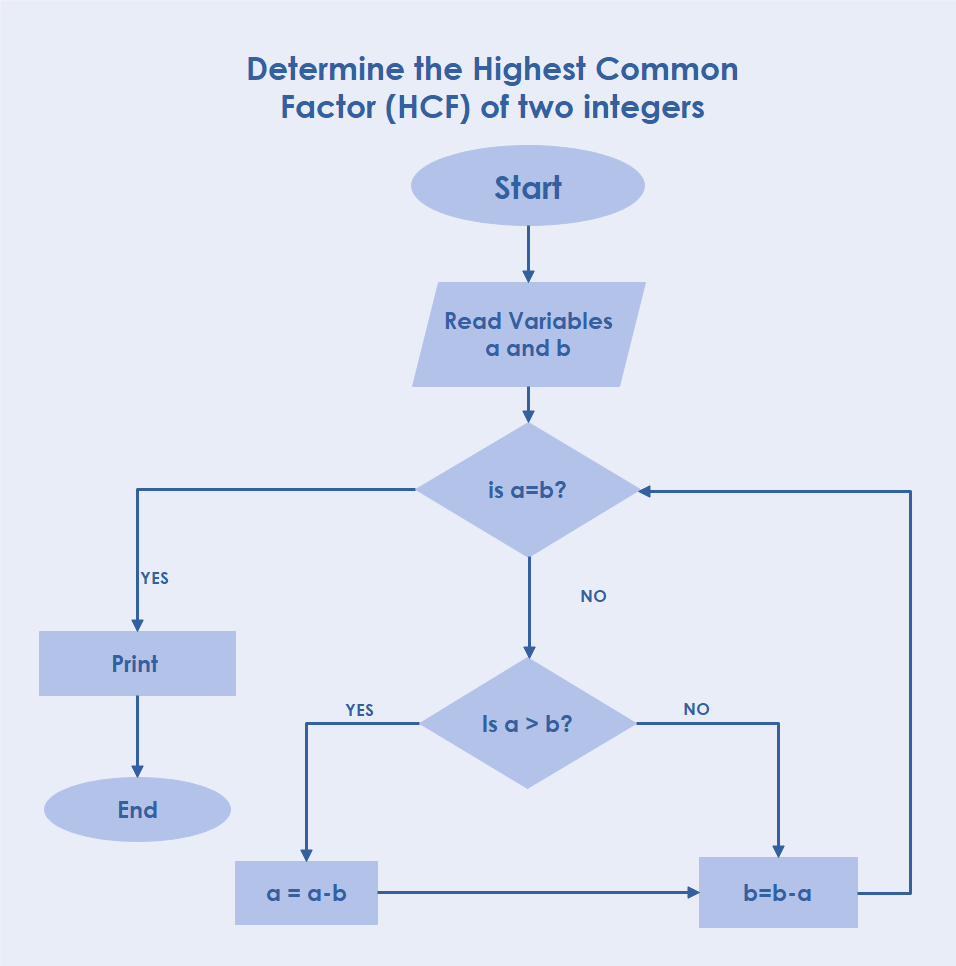#### Part 4: How to Create a Flowchart for Algorithm?

Step 2: Click on the New menu and select the blank template with a + sign to create a flowchart from scratch. However, you can also benefit from the pre-existing templates by clicking the Flowcharts menu.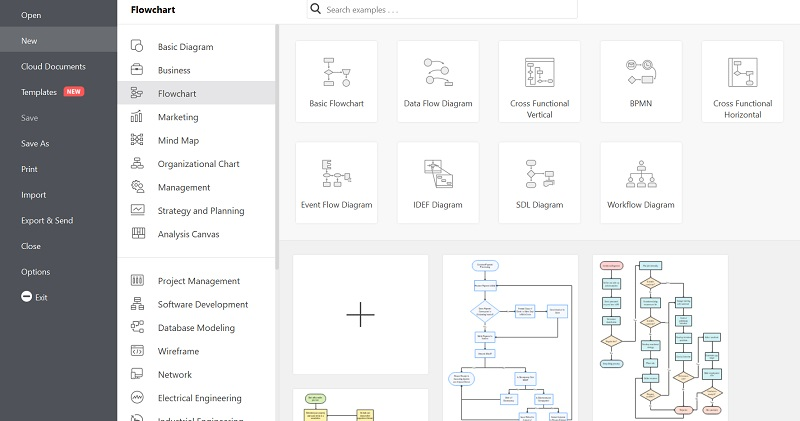Step 3: You will be directed to a workspace. In the left pane, select [Symbol Library] followed by [Basic Flowchart Shapes] to add these shapes to the left library pane.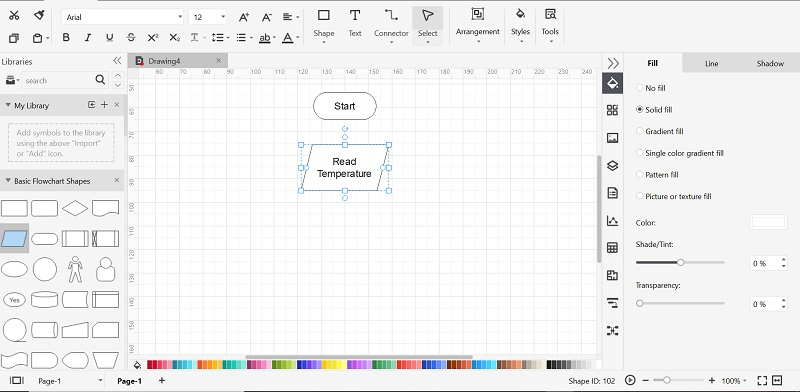Step 4: Insert the [Start] symbol in the workspace to get started. Then drag and drop other symbols you need to make the main body. To join all your symbols, navigate to the toolbar and select [Connector]. This will add flow lines between all your shapes and symbols.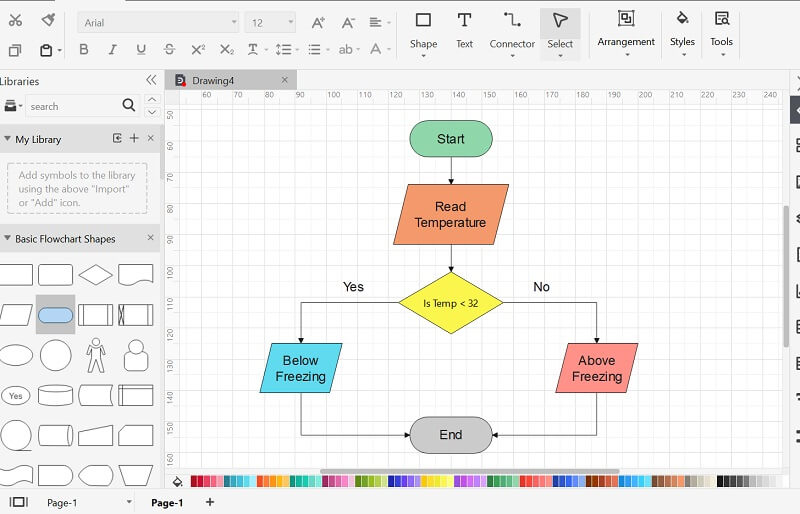Step 5: After you have created the web for your chart, you can customize and edit it using plenty of editing tools available.

Step 6: After you are done, save it as an EdrawMax file by going to [File] and select [Save]. If you want to export the file, go to [File], click [Export & Send], select the file type, and press [OK].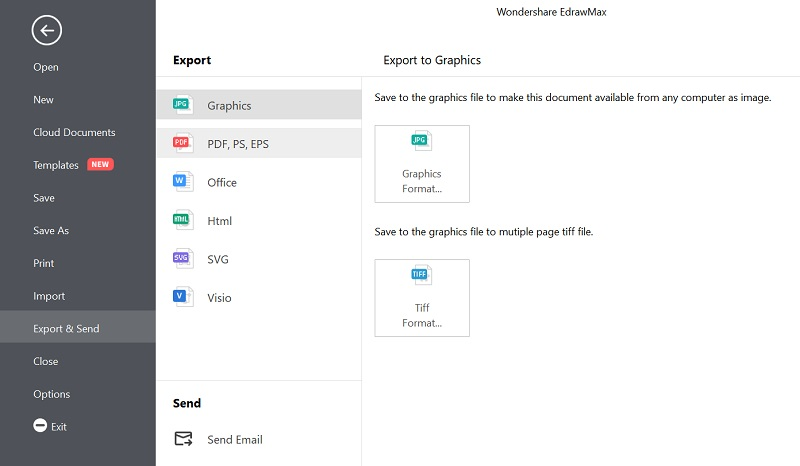#### Part 5: Conclusion

Flowcharts are a significant way of understanding different processes and their requirements. The graphical representation will aid you in linking different elements and decisions.

EdrawMax is an impressive software for quickly drawing professional flowcharts and diagrams. The wide array of tools and symbols make everything a breeze. You can easily craft the simplest to the most complex diagrams in no time at all. Give Edraw Max a shot and create professional-grade drawings effortlessly.

## Get Started! You Will Love This Easy-To-Use Diagram Software

EdrawMax is an advanced all-in-one diagramming tool for creating professional flowcharts, org charts, mind maps, network diagrams, UML diagrams, floor plans, electrical diagrams, science illustrations, and more. Just try it, you will love it!